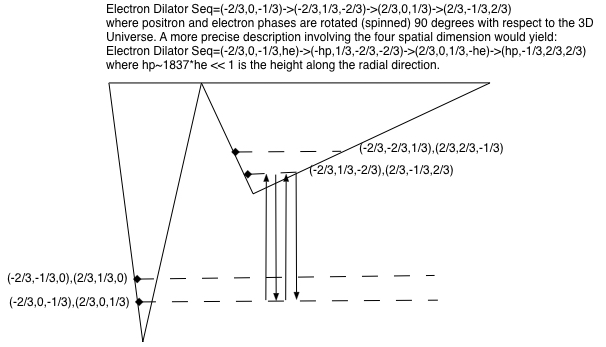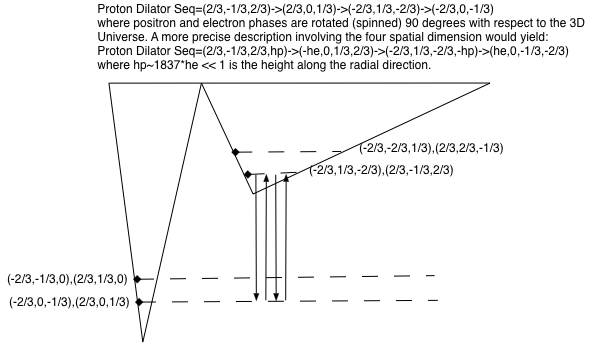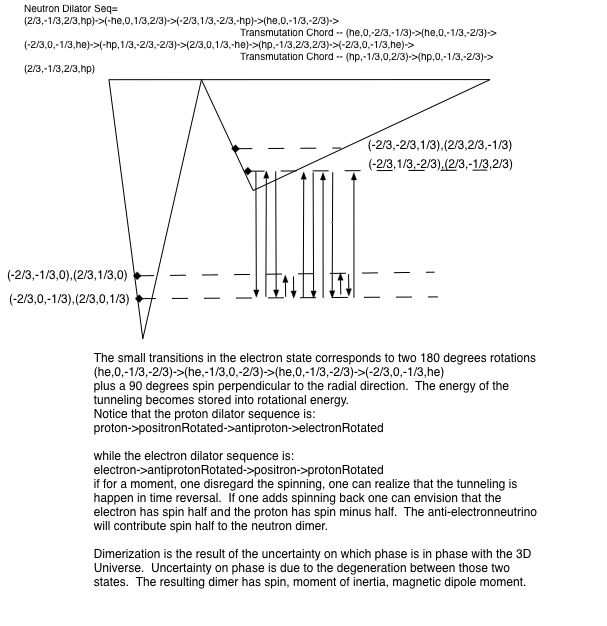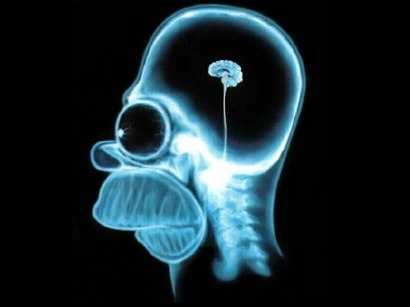# Human Nature

After you watch this amazing video about the beauty of our Planet, you can take a look at the small progress I made on defining the base classes on the Hypergeometrical Standard Model in our collaborative project.

I aimed at collaboration since I know that there isn't any stronger force than the pleasure of discovery.... so I tried to make discovery a group effort.

On the other hand, the initial classes are really difficult to design and it is understandable that most people don't even know where to begin.

The base classes are:

• Dilator
• Unit - capable of plotting itself
• Hyperon - capable of addition to other hyperons and neutrinos

The Dilator is basically the Fundamental Dilator and provides the four 4D ellipsoids associated with the Fundamental Dilator Coherence. It then loads those into Unit classes for drawing.  I might derive the dilator from Unit later.  I've just have little time to do this kind of work now.

Currently I am building the Hyperon class to append dilators and pay attention to the effect of neutrinos (in fact, half-neutrinos) on its evolution.  A half electron-antineutrino corresponding to (0,-1/3,1/3) will keep the latest dilator state, rotate it around the x-axis while the spinning continues.  This will bring the next phase to be the spatially rotated version of the prior dilator state, thus transmutating one particle into another.

This is necessary since in my theory a neutron is a composite dimer. Each moiety containing electron/half-anti-electronneutrino/proton/half-anti-electronneutrino.

Similarly, a pion minus is an electron/half-muonneutrino/positron/half-muon-neutrino.  This hyperon, in principle, could be modeled as electron/half-muonneutrino/proton/half-muon-neutrino since that would yield the correct charge.  I discarded that option due to the decaying reactions and the higher mass.  One can imagine a particle family in which a FatPionPlus would exist and be made of:

electron/n-half-muonneutrino/proton/n-half-muon-neutrino

decaying along a different path and emitting many muon neutrinos.

In terms of metric displacement one can write:

half-electronNeutrino= (0,1/3,-1/3,)

electron = (-2/3,-1/3,0,he)  where he is a small value or thickness along the radial direction (perpendicular to the 3D Universe).

proton = (2/3,2/3,-1/3,hp)  where hp is a small value or thickness along the radial direction (perpendicular to the 3D Universe)

he/hp=mp/me = 1836.15267389

The interpretation on these 4-vectors is metric deformation displacement.  Lets consider a cartesian four-dimensional space. To make life simpler, lets consider a hyper cylinder where the 3D part is a 3D sphere of unit radius and height along the radial direction is also unit (1).

Now let's overlay over that sphere a small deformation alpha * proton (for instance).  That means that the deformed sphere is now a 3D ellipsoid with axes

(1+alpha*2/3, 1+alpha*2/3, 1-apha*1/3).  Alpha exact value is irrelevant, but it is very small.  The displacement volume due to this metric deformation is the product (1+alpha*2/3) * (1+alpha*2/3*(1-apha*1/3) - 1.  Considering alpha very small (disregarding second order contributions in alpha), the resulting difference is alpha.  Similarly, the resulting metric displacement in the electron is -alpha and zero for the neutrino.

The axes of each dilator has to be defined in a consistent manner spattially and with respect to spin.

`def getDilatorSequence(particle=0, axis=0, spin='half'):    hp = 1e-9    he = hp * 1837    ident = np.identity(4)    rotate = spinMe(axis=axis)    rotationMatrix = [ident,rotate,ident,rotate]    # Particle Definition    protonCoeff = np.array([2/3, 2/3, -1/3,hp]).T    electronCoeff = np.array([-2/3,-1/3,0,he]).T    positronCoeff = np.array([2/3,1/3,0, -he]).T    antiprotonCoeff = np.array([-2/3, -2/3, 1/3,-hp]).T    halfelectronAntiNeutrinoCoeff=np.array([0,1/3,-1/3,he]).T    if(spin=='half'):        listA = [protonCoeff, positronCoeff,antiprotonCoeff, electronCoeff,protonCoeff, positronCoeff,antiprotonCoeff, electronCoeff]    else:        listA=[protonCoeff,electronCoeff ,antiprotonCoeff, positronCoeff,protonCoeff,electronCoeff ,antiprotonCoeff,positronCoeff ]    listA = listA[particle:(particle+5)]    dilatorSequence = [np.dot(rotationMatrix[i],listA[i]) for i in np.arange(4)]    A = [x[0:4] for x in dilatorSequence]    return A`

This method yields the 4D ellipsoid sequence.  Here we used arbitrarily

`hp = 1e-9he = hp * 1837`

proton dilator sequence:

• array([ 6.66666667e-01, 6.66666667e-01, -3.33333333e-01,1.00000000e-09]) =(2/3,2/3,-1/3, hp),
• array([ -1.83700000e-06, 3.33333333e-01, 0.00000000e+00, 6.66666667e-01]) = (-he,1/3,0,2/3),
• array([ -6.66666667e-01, -6.66666667e-01, 3.33333333e-01,-1.00000000e-09]) =(-2/3,-2/3,1/3,-hp),
• array([ 1.83700000e-06, -3.33333333e-01, 0.00000000e+00,-6.66666667e-01]) = (he,-1/3,0,-2/3),

The first three numbers corresponds to what can be seen in the 3D Universe.  Notice that the dilator states are: proton, rotatedPositron,anti-Proton, rotatedElectron. Notice that if one allows the last state to rotate and if instead of tunneling it were to absorb a half-anti-electron-neutrino, the resulting new state would be:

• array([-6.66666667e-01, -3.33333333e-01, 0.00000000e+00,  1.83700000e-06, ]) + (0,1/3,-13)= (-2/3,-1/3,0,he) +(0,1/3,-13,0)   = (-2/3,0, -1/3,he)

The absorption of a half electron neutrino transmutates a proton into an electron while rotating it around the x-axis in this case.

This means that the subsequence tunnelings and spinning will lead to an electron dilator sequance, at the end of which another half-anti-electronneutrino would be absorbed and the dilator would transmutate back into a proton with the right orientation.

The two half-anti-electronneutrinos carry the centrifugal energy associated with the neutron being a rotating dimer.  Since we know that motion has to do with the relaxation of the local metric, each moiety of the dimer land in a tilted local metric (non-relaxed space) and like the Silver Surfer, rotates around the radial direction.  This gives the neutron its spin and magnetic moment.  The rotation of these charges creates a magnetic dipole and a moment of inertia. All these properties have to be matched in the calculation of the angle mismatch associated with the half-anti-electronneutrino.

Below we present the 'energy level' diagrams.  Energy levels is enclosed between quote marks because degenerated deformation states can become undegenerated because of dynamics (stated differs from each other by ellipsoid orientation within the 3D Universe).

Below is the Electron Energy Diagram and Coherence:Below is the Proton Energy Diagram and Coherence:Below is the Neutron Energy Diagram and Coherence:The neutron dimer means that two dilator sequences rotate around a common center which is in the center between the two dilator and not in the center of mass between a proton and a electron.  Also, the rotation is around an axis perpendicular to the 3D Universe, so it is clearly not something people think about everyday, so I am sure it will take a little time for people to understand...:)So the topology of a neutron is a rotating segment.  The topology of a pion minus/plus is a rotating triangle.  The topology of a pion zero is a rotating square....:) and so on and so forth.

The phase difference between spinning and rotating with the energy associated with the tunneling creates a locally tilted metric (unrelaxed local metric).  The level of tilting maps into the inverse of the lifetime of the particle.  Zero tilting implies infinite lifetime (proton, electron,positron,antiproton).  Everything else contains transmutation chords and have a finite lifetime.

Cheers,

MP

PS.  I reversed the potential wells to reflect that protons have a larger 3D footprint, and so they should be subject to a broader potential well.  Normally, broader wells are in excited states, so it seems that the electron state occupies the lowest energy level of all...:) (this is a half-joke since they are all part of the same coherence, just different phases).  Also, one has to acknowledge that the anti-electronneutrino could be a rotation in the electron or in the proton state, so the assignment is not perfectly defined at this posting.  Normally the Physics is very important.  In this case, the Physics can be thought as the final dephasing between tunneling and spinning. The angle (thus the angular velocity) is what really matters.

Currently unrated

### New Comment

required

required (not published)

optional

required